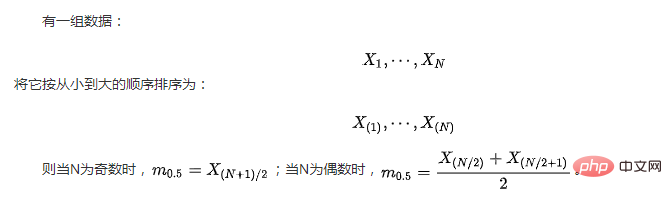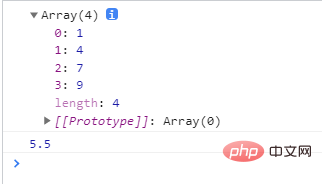# JavaScript如何获取数字数组的中位数（两种方法）```<!DOCTYPE html>
<html lang="en">

<meta charset="UTF-8" />
<title></title>
<script>
function medianof2Arr(arr1) {
var concat = arr1;
concat = concat.sort(
function (a, b) { return a - b });

console.log(concat);
var length = concat.length;

if (length % 2 == 1) {

// 如果长度是奇数
console.log(concat[(length / 2) - .5])
return concat[(length / 2) - .5]

}
else {
console.log((concat[length / 2]
+ concat[(length / 2) - 1]) / 2);

return (concat[length / 2]
+ concat[(length / 2) - 1]) / 2;
}
}

arr1 = [1, 4, 7, 9]

medianof2Arr(arr1)
</script>
<body>
</body>

</html>``````<!DOCTYPE html>
<html lang="en">

<meta charset="UTF-8" />
<title></title>
<script>
function median_of_arr(arr) {
const middle = (arr.length + 1) / 2;

// 避免在排序时发生变异
const sorted = [...arr].sort((a, b) => a - b);
const isEven = sorted.length % 2 === 0;

return isEven ? (sorted[middle - 1.5]
+ sorted[middle - 0.5]) / 2 :
sorted[middle - 1];
}
var arr = [1, 4, 7, 9];
console.log(median_of_arr(arr));

</script>
<body>
</body>

</html>```### 全部评论我要评论

• 取消发布评论发送
• 1/1